Assumptions in the theory of HMMsNext: Three basic problems of Up: Hidden Markov Models Previous: Definition of Hidden Markov

# Assumptions in the theory of HMMs

For the sake of mathematical and computational tractability, following assumptions are made in the theory of HMMs.

(1)The Markov assumption
As given in the definition of HMMs, transition probabilities are defined as,In other words it is assumed that the next state is dependent only upon the current state. This is called the Markov assumption and the resulting model becomes actually a first order HMM.
However generally the next state may depend on past k states and it is possible to obtain a such model, called an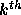order HMM by defining the transition probabilities as follows.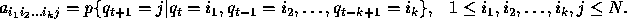But it is seen that a higher order HMM will have a higher complexity. Even though the first order HMMs are the most common, some attempts have been made to use the higher order HMMs too.

(2)The stationarity assumption
Here it is assumed that state transition probabilities are independent of the actual time at which the transitions takes place. Mathematically,for any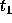and.

(3)The output independence assumption
This is the assumption that current output(observation) is statistically independent of the previous outputs(observations). We can formulate this assumption mathematically, by considering a sequence of observations,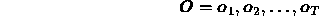. Then according to the assumption for an HMM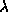,However unlike the other two, this assumption has a very limited validity. In some cases this assumption may not be fair enough and therefore becomes a severe weakness of the HMMs.